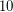# Sum of prime and semiprime conjecture

 Importance: Medium ✭✭
 Author(s): Geoffrey Marnell
 Subject: Number Theory
 Keywords: prime semiprime
 Posted by: princeps on: March 23rd, 2012
Conjecture   Every even number greater thancan be represented as the sum of an odd prime number and an odd semiprime .

## Bibliography

*[M] Geoffrey R. Marnell, "Ten Prime Conjectures", Journal of Recreational Mathematics 33:3 (2004-2005), pp. 193--196.

* indicates original appearance(s) of problem.

### surely that's Chen's theorem

Every sufficiently large even number is the sum of either 2 primes or a prime and a semiprime.

### Yes, apart from the

Yes, apart from the "sufficiently large" and allowing prime + prime as well as prime + semiprime. The parity problem makes the latter hard, but some progress has been made, see arXiv:math/0609615 and arXiv:0803.2636. (The key progress is their use of E2 = semiprimes rather than P2 = primes or semiprimes.)